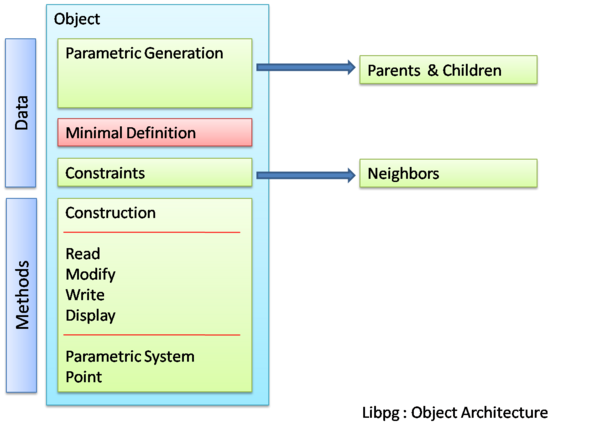# Difference between revisions of "Libpg : A parametrics/constraint library"This page contains the design document for an enhancement or feature. The design should be considered a work in progress and may not represent the final design. As this is a collaborative design, contributions and participation from other developers and users is encouraged. Use the discussion page for providing comments and suggestions.

# libpg : A parametrics/ constraint library

Proposed sytem for parametrics and constraint implementation by Dawn Thomas (homovulgaris)

Provision for Parametrics and constraints greatly improves the performance of a Computer Aided Design System both in terms of geometry generation as well as analysis. Considering the unix model of division into multiple tools and libraries for individual functions as well as the fact that parametric/constraint functionalities are not critical elements in terms of geometry generation and raytracing, a logical solution would be the implemenation of a separate library (libpg)

## Function

1. Creation & Modification of parameter-based geometry objects
2. Creation of constraints between geometry objects
3. Creation & Storage of Parametric Objects (non-geometric)
4. Modification of geometry objects in .g files to indicate parametric nature

Part 1 would include building parametric objects based on existing non-parametric geometry objects as well as generation of purely parametric geometry. libpg acts as a library which sits above librt using the librt methods for creation and modification of geometry

## Architecture

A constraint system can be looked upon as a system of variables, their associated domains and set of constraints/relationships between the variables.

Fundamentally this can be visualised using a constraint network which is a 3-tuple.For a mathematical review please see ( Constraint Networks by Dawn Thomas ). Further we can have a graph based visualization of the same using vertices as variables and edges connecting vertices as constraints. It would be intuitive only for networks having binary (between two variables) or unary (constraints on self) constraints. Otherwise, one has to visualize hypergraphs which contain hyperedges which is basically a line connecting multiple vertices for example.

Implementation of the above constraint network in the BRL-CAD system, involves 3 fundamental aspects.

1. Domain extraction 2. Solution 3. Geometry updation

Prerequisites for such a state is of course

1."Handle" generation and storage 2. Updated geometry storage

A typical constraint solution operation is shown below.

## Object Architecture

An object oriented method of implementation would be the creation of a mixed ( in the sense that they contain both geometric and non-geometric information ) object. The object architecture is as shown below which shows the data types as well as the public and private methods.### Parametric Geometric Objects

From an Object Oriented point of view the major advantages is in terms of the structuring of data and in particular the system of calling methods or procedures. In effect each object knows how to evaluate equations within its space. For example a line or a curve knows ( has a method to ) calculate and return the coordinates of a point with a certain parameter value in its space ( a point at 0.6 ratio of length of the curve) With this value ( coordinates) thus returned and with the existing knowledge ( parent: curveid , parameter value:0.6 ) a parametric point object has the methods to instantiate it and store it.

### Constraint Objects

The above method is efficient and useful only in the generation, modification and analysis of new geometry based on existing parametric/ non-parametric geometry. The evaluation method for constraints between already existing independent geometry would require special constraint objects. The effective difference between these objects and the earlier arises from the fact that generation of the parametric geometric objects depend on parametrics whereas constraints may or may not be feasible and thus evaluation would result in multiple solutions and they represent relationship between two independently defined elements ( parametrically or not )

### Evaluation System

It would be ideal to provide both analytical and numeric evaluation methods the second one being of primary importance in terms of constraint based calculations. Considering the standard methods of parametrization ( see Enumeration below ) I think the implementation of an analytic solving system would be easier. Though for the solution of more complex equation as well as majority of constraints , libpg will have to provide support for numerical solutions.

## Enumeration of Parameters and Constraints

Various standard methods of parametrics and constraints available in CAD Programs ( CATIA as a case study )

### Parametrics

1. Point
1. Coordinates
2. On Curve
3. On Plane
4. On Surface
5. Circle/Sphere Centre
6. Tangent on Curve
7. Between (2 points)
8. Extremum
9. Extremum Polar
2. Line
1. Point-Point
2. Point-Direction
3. Angle/Normal to the curve
4. Tangent to the Curve
5. Normal to the surface
6. Bisecting
3. Plane
1. Offset from plane
2. Parallel through Point
3. Angle/Normal to Plane
4. Three points
5. Two Lines
6. Point and Line
7. Planar Curve
8. Normal to Curve
9. Tangent to Surface
10. Equation
11. Mean through points
4. Axis
5. Polyline
6. Circle
7. Corner
8. Connect Curve
9. Conic
10. Spline
11. Helix
12. Spiral

### Constraints

1. Distance
2. Length
3. Angle
5. Semimajor axis
6. Semiminor axis
7. Symmetry
8. Midpoint
9. Equidistant point
10. Fix
11. Coincidence
12. Cocentricity
13. Tangency
14. Parallelism
15. Perpendicular
16. Horizontal
17. Vertical
18. Angle with axis